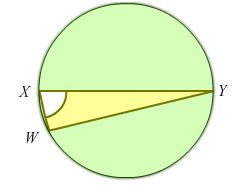An Angle Discovery!

Geometry Level 4Given that $XY$ is the diameter of the circle, where $\triangle WXY$ is enclosed in the circle and that the ratio $\frac { Area\quad of\quad circle }{ Area\quad of\quad \triangle WXY } =2\pi$.

Find the acute angle $WXY$. Giving your answer in degrees.

×